Publicité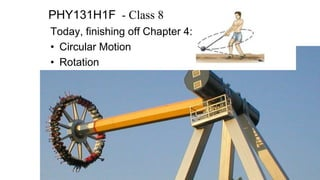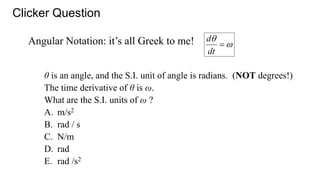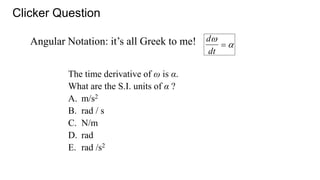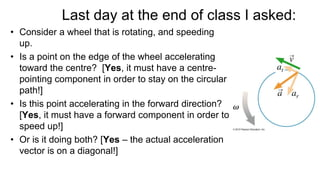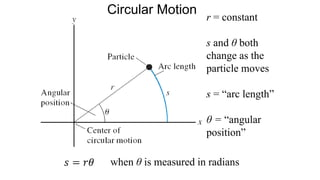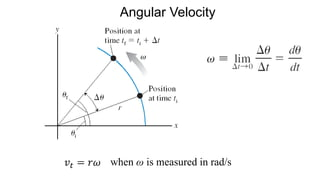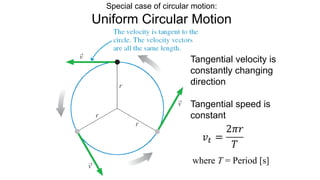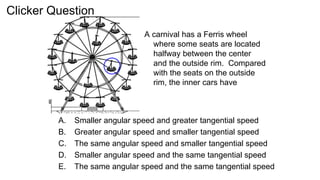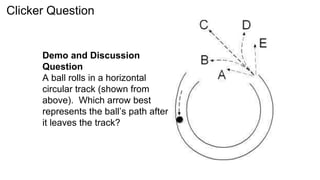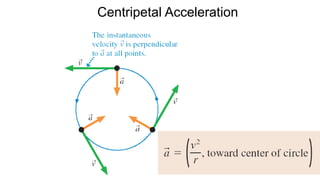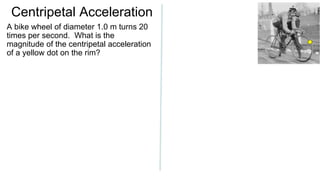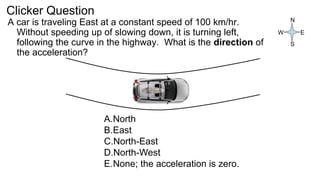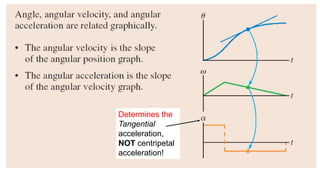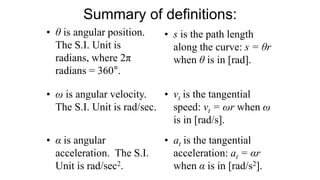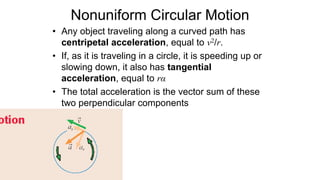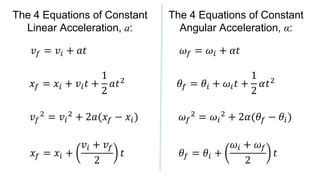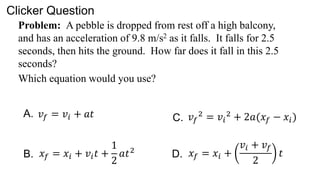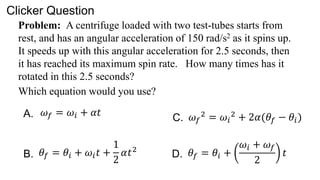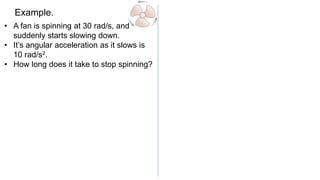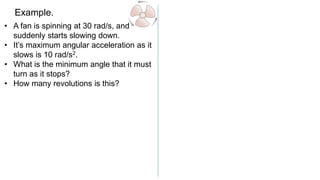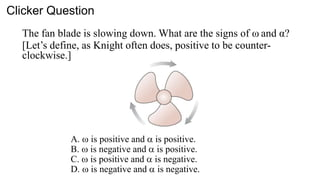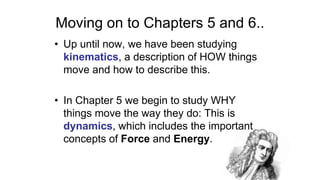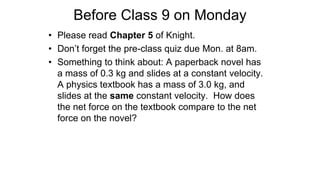1 sur 23
Publicité

### rotation ppt

1. PHY131H1F - Class 8 Today, finishing off Chapter 4: • Circular Motion • Rotation
2. Angular Notation: it’s all Greek to me! θ is an angle, and the S.I. unit of angle is radians. (NOT degrees!) The time derivative of θ is ω. What are the S.I. units of ω ? A. m/s2 B. rad / s C. N/m D. rad E. rad /s2    dt d Clicker Question
3. Angular Notation: it’s all Greek to me! The time derivative of ω is α. What are the S.I. units of α ? A. m/s2 B. rad / s C. N/m D. rad E. rad /s2  d dt   Clicker Question
4. Last day at the end of class I asked: • Consider a wheel that is rotating, and speeding up. • Is a point on the edge of the wheel accelerating toward the centre? [Yes, it must have a centre- pointing component in order to stay on the circular path!] • Is this point accelerating in the forward direction? [Yes, it must have a forward component in order to speed up!] • Or is it doing both? [Yes – the actual acceleration vector is on a diagonal!]
5. Circular Motion r = constant s and θ both change as the particle moves s = “arc length” θ = “angular position” when θ is measured in radians
6. Angular Velocity when ω is measured in rad/s
7. Special case of circular motion: Uniform Circular Motion Tangential velocity is constantly changing direction Tangential speed is constant where T = Period [s]
8. A carnival has a Ferris wheel where some seats are located halfway between the center and the outside rim. Compared with the seats on the outside rim, the inner cars have A. Smaller angular speed and greater tangential speed B. Greater angular speed and smaller tangential speed C. The same angular speed and smaller tangential speed D. Smaller angular speed and the same tangential speed E. The same angular speed and the same tangential speed Clicker Question
9. Demo and Discussion Question A ball rolls in a horizontal circular track (shown from above). Which arrow best represents the ball’s path after it leaves the track? Clicker Question
10. Centripetal Acceleration
11. Centripetal Acceleration A bike wheel of diameter 1.0 m turns 20 times per second. What is the magnitude of the centripetal acceleration of a yellow dot on the rim?
12. A car is traveling East at a constant speed of 100 km/hr. Without speeding up of slowing down, it is turning left, following the curve in the highway. What is the direction of the acceleration? N A.North B.East C.North-East D.North-West E.None; the acceleration is zero. E S W Clicker Question
13. Determines the Tangential acceleration, NOT centripetal acceleration!
14. Summary of definitions: • θ is angular position. The S.I. Unit is radians, where 2π radians = 360°. • s is the path length along the curve: s = θr when θ is in [rad]. • ω is angular velocity. The S.I. Unit is rad/sec. • vt is the tangential speed: vt = ωr when ω is in [rad/s]. • α is angular acceleration. The S.I. Unit is rad/sec2. • at is the tangential acceleration: at = αr when α is in [rad/s2].
15. Nonuniform Circular Motion • Any object traveling along a curved path has centripetal acceleration, equal to v2/r. • If, as it is traveling in a circle, it is speeding up or slowing down, it also has tangential acceleration, equal to rα • The total acceleration is the vector sum of these two perpendicular components
16. The 4 Equations of Constant Linear Acceleration, a: 𝑣𝑓 = 𝑣𝑖 + 𝑎𝑡 𝑥𝑓 = 𝑥𝑖 + 𝑣𝑖𝑡 + 1 2 𝑎𝑡2 𝑣𝑓 2 = 𝑣𝑖 2 + 2𝑎(𝑥𝑓 − 𝑥𝑖) 𝑥𝑓 = 𝑥𝑖 + 𝑣𝑖 + 𝑣𝑓 2 𝑡 𝜔𝑓 = 𝜔𝑖 + 𝛼𝑡 𝜃𝑓 = 𝜃𝑖 + 𝜔𝑖𝑡 + 1 2 𝛼𝑡2 𝜔𝑓 2 = 𝜔𝑖 2 + 2𝛼(𝜃𝑓 − 𝜃𝑖) 𝜃𝑓 = 𝜃𝑖 + 𝜔𝑖 + 𝜔𝑓 2 𝑡 The 4 Equations of Constant Angular Acceleration, α:
17. Problem: A pebble is dropped from rest off a high balcony, and has an acceleration of 9.8 m/s2 as it falls. It falls for 2.5 seconds, then hits the ground. How far does it fall in this 2.5 seconds? Which equation would you use? Clicker Question 𝑣𝑓 = 𝑣𝑖 + 𝑎𝑡 𝑥𝑓 = 𝑥𝑖 + 𝑣𝑖𝑡 + 1 2 𝑎𝑡2 𝑣𝑓 2 = 𝑣𝑖 2 + 2𝑎(𝑥𝑓 − 𝑥𝑖) 𝑥𝑓 = 𝑥𝑖 + 𝑣𝑖 + 𝑣𝑓 2 𝑡 A. B. C. D.
18. Problem: A centrifuge loaded with two test-tubes starts from rest, and has an angular acceleration of 150 rad/s2 as it spins up. It speeds up with this angular acceleration for 2.5 seconds, then it has reached its maximum spin rate. How many times has it rotated in this 2.5 seconds? Which equation would you use? Clicker Question A. B. C. D. 𝜔𝑓 = 𝜔𝑖 + 𝛼𝑡 𝜃𝑓 = 𝜃𝑖 + 𝜔𝑖𝑡 + 1 2 𝛼𝑡2 𝜔𝑓 2 = 𝜔𝑖 2 + 2𝛼(𝜃𝑓 − 𝜃𝑖) 𝜃𝑓 = 𝜃𝑖 + 𝜔𝑖 + 𝜔𝑓 2 𝑡
19. Example. • A fan is spinning at 30 rad/s, and suddenly starts slowing down. • It’s angular acceleration as it slows is 10 rad/s2. • How long does it take to stop spinning?
20. Example. • A fan is spinning at 30 rad/s, and suddenly starts slowing down. • It’s maximum angular acceleration as it slows is 10 rad/s2. • What is the minimum angle that it must turn as it stops? • How many revolutions is this?
21. The fan blade is slowing down. What are the signs of ω and α? [Let’s define, as Knight often does, positive to be counter- clockwise.]  is positive and  is positive. B.  is negative and  is positive. C.  is positive and  is negative. D.  is negative and  is negative. Clicker Question
22. Moving on to Chapters 5 and 6.. • Up until now, we have been studying kinematics, a description of HOW things move and how to describe this. • In Chapter 5 we begin to study WHY things move the way they do: This is dynamics, which includes the important concepts of Force and Energy.
23. Before Class 9 on Monday • Please read Chapter 5 of Knight. • Don’t forget the pre-class quiz due Mon. at 8am. • Something to think about: A paperback novel has a mass of 0.3 kg and slides at a constant velocity. A physics textbook has a mass of 3.0 kg, and slides at the same constant velocity. How does the net force on the textbook compare to the net force on the novel?
Publicité